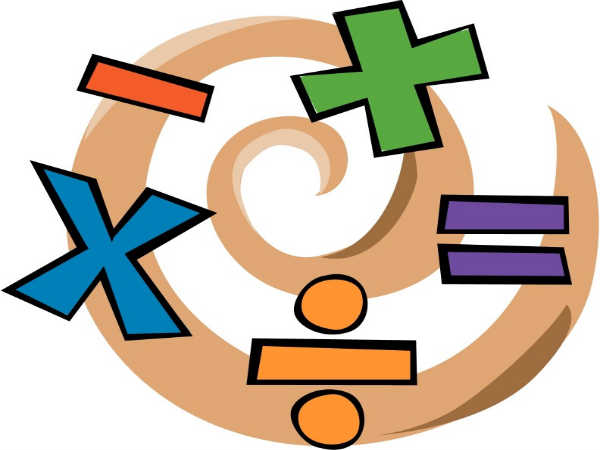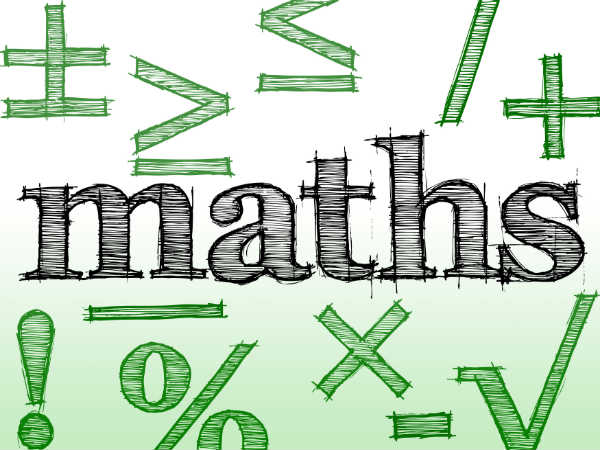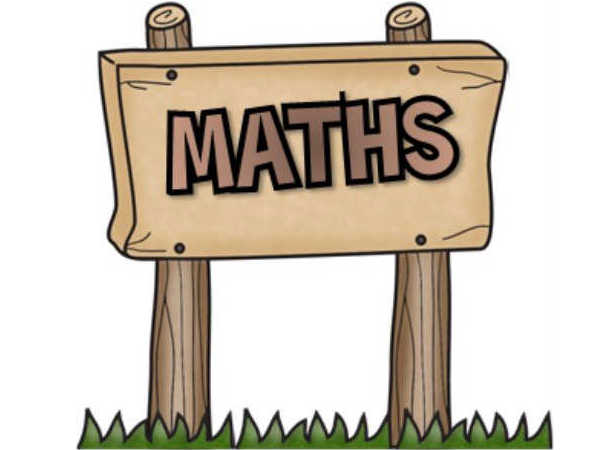# World Maths Day: 20 Interesting and Amazing Facts About Maths

By Staff

Words such as formula, equation and calculation sounds boring for those who hate Maths as a subject, whereas it is fun for those who have keen interest towards solving equations/problems.

October 14th is celebrated as World Maths Day. Let us know some interesting and amazing facts about Mathematics.

1. Zero ( 0 ) is the only number which can not be represented by Roman numerals.

2. What comes after a million, billion and trillion? A quadrillion, quintillion, sextillion, septillion, octillion, nonillion, decillion and undecillion

3. Plus (+) and Minus (-) sign symbols were used as early as 1489 A.D

4. 2 and 5 are the only primes that end in 2 or 5

5. An icosagon is a shape with 20 sidesMersal Mathematics: 5 Shortcuts for Multiplication!

6. Among all shapes with the same perimeter a circle has the largest area.

7. Among all shapes with the same area circle has the shortest perimeter

8. 40 when written "forty" is the only number with letters in alphabetical order, while "one" is the only one with letters in reverse order

9. 'FOUR' is the only number in the English language that is spelt with the same number of letters as the number itself

10. From 0 to 1,000, the letter "A" only appears in 1,000 ("one thousand")

11. 12,345,678,987,654,321 is the product of 111,111,111 x 111,111,111. Notice the sequence of the numbers 1 to 9 and back to 1.7 Mathematics Shortcuts That Can Blow You Down

12. Have you ever noticed that the opposite sides a die always add up to seven (7)

13. Trigonometry is the study of the relationship between the angles of triangles and their sides

14. Abacus is considered the origin of the calculator

15. Here is an interesting trick to check divisibility of any number by number 3.A number is divisible by three if the sum of its digits is divisible by three (3)

16. Do you know the magic of no. nine (9)? Multiply any number with nine (9 ) and then sum all individual digits of the result (product) to make it single digit, the sum of all these individual digits would always be nine (9).

17. If you add up the numbers 1-100 consecutively (1+2+3+4+5...) the total is 5050

18. A 'jiffy' is an actual unit of time for 1/100th of a second

19. Have you heard about a Palindrome Number? It is a number that reads the same backwards and forward, e.g. 12421

20. Have you heard about Fibonacci? It is the sequence of numbers wherein a number is the result of adding the two numbers before it! Here is an example: 1, 1, 2, 3, 5, 8, 13, 21, 34, and so onMaths Aptitude Questions Types For Entrance Exams and Recruitment Preparation

Subscribe Now

--Or--
Select a Field of Study
Select a Course
Select UPSC Exam
Select IBPS Exam
Select Entrance Exam